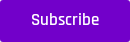Data Preparation

# Train VS Validation VS Test Sets: Harnessing the Power of Three

Train, validation, and test sets. Is this the normal way to split your data? What could possibly go wrong if one of them is omitted?

Even if you are a beginner in machine learning, you would know that you have to partition your data into training and test sets. Speaking in layman's terms, the training set is used to help the model learn the hidden trends and patterns in the data using labeled data, and the test set is used to evaluate how well the model predicts on completely unseen data.

However, there is widespread confusion as to why a validation set is necessary. Is it part of the training or the testing phase? What could possibly go wrong if the validation set is omitted?

###### Just getting started with AI/ML? Get the basics of Machine Learning in this article.

This article aims to address this problem. Let’s see what each set is used for in machine learning, and the significance of data splitting into each set will become clearer.

## The Training Set

It is the set of data used to train the model. For example, if we are training a neural network, we would use the training set to adjust the weights of the neurons to minimize the loss function.

## The Validation Set

It is the set of data used to fine-tune the hyper-parameters, do regularization and then choose the best model. It is sometimes also referred to as the holdout set. Going back to neural networks, we would use the validation set to tune the number of hidden layers, learning rate, activation function, etc.

One of the major reasons why we need a validation set is to ensure that our model is not over-fitting the data in the training set. This is made possible since the trained model has not seen the samples present in the validation set. Hence, if the model is performing well on the training set but not on the validation set, we say the model is over-fitting and will, consequently, fail to generalize well on unseen data.

## The Test Set

It is the set of data used to get an unbiased estimate of the model performance after its hyper-parameters have been tuned. The test set is unlabelled, as opposed to the training and validation sets which are labeled so we can see the evaluation metrics. The model should not have the test data before, even indirectly - i.e. the test set should never be used to do any training. It is meant to assess the final performance of the model. It is similar to how the model would behave when it is deployed into production to make predictions on real data.

Generally, 70% of the data can be used for training and 15% each for validation and testing. However, this is not a hard and fast rule and if you have a huge data set, you can use almost all of it for training and leave only a small portion for validation and testing.

### SummaryYou should use the training data to train your model, the validation data to tune the hyper-parameters and regularize to choose the best model, and the test data to evaluate the performance of your final model. Omitting the validation set would make the model susceptible to over-fitting and hence will not generalize well on unseen data.

#### References: